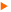TutorialThis tutorial provides some lessons to learn how to use and develop codes using . The tutorial is constructed as a set of problems with progressive complexity. We start by considering a one-dimensional problem and then different types of problems with varying difficulties.
This set of lessons can be advantageously be used for teaching purposes.
Note that comments on code lines are not reported from one lesson to another. If something is not clear in Lesson n, just look at Lesson n-1, may be you will find some explanation, if not, see n-2 and henceforth.Lesson 1: A 1-D problemLesson 2: A 2-D diffusion problemLesson 3: A 2-D diffusion problem using an iterative solverLesson 4: A time dependent problemLesson 5: An optimization problemLesson 6: Using parameter data fileLesson 7: Using mesh generatorLesson 8: Using file converters